We will now discuss the Phi Scaling Angle, Fibonacci Circles and Roots, the Golden Triangle, Golden Rectangles and the Golden Angle.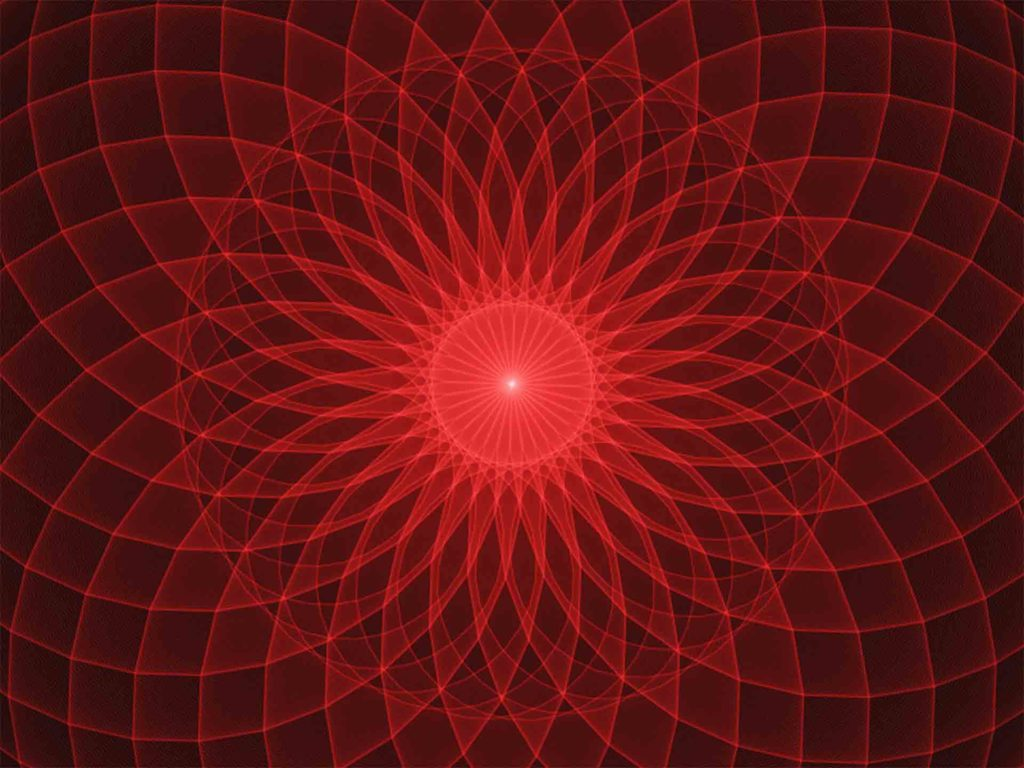# Phi Scaling Angle

The Phi Scaling angle is the angle of expansion.

When a set of circles (or spheres) with diameters expanding by Fibonacci values, are placed tangential to one another in a straight line the angle between the horizontal line at the bottom of each circle and the line that touches each circle’s “upper” surface point is the phi scaling angle.1

It is approximately 27.5º (27.30916949195…).

The center angle that intersects each circle’s center point is 13.75 (1/10 of 137.5).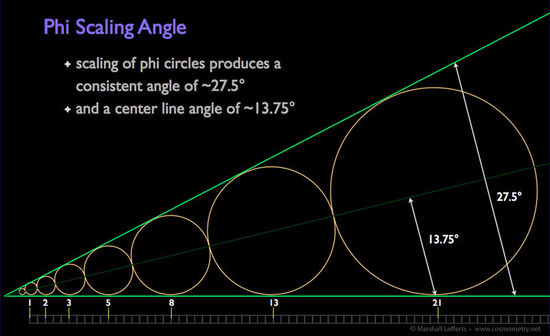Credit: http://cosmometry.net/phi-scaling-angle

This is seen in the growth of a spruce tree; sea shells; v-shaped flocks of birds; sand patterns left by waves; weave patterns in some tree bark, and other natural phenomenon.

Reference Construction Lesson #62: Phi Scaling Angle & Fibonacci Circles.

# Gnomonic Growth & Gnomons

Gnomons are things that suffer no change other than magnitude when they grow.

This concept was discovered by Aristotle, as far as we know.

A gnomon is any figure, which, when added to another figure, leaves the resultant figure similar to the original.In geometry, a gnomon is a plane figure formed by removing a similar parallelogram from a corner of a larger parallelogram; or, more generally, a figure that, added to a given figure, makes a larger figure of the same shape.

This concept is also seen in square and triangular numbers (as well as rectangular and cubic numbers):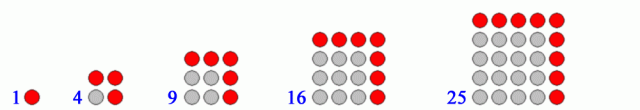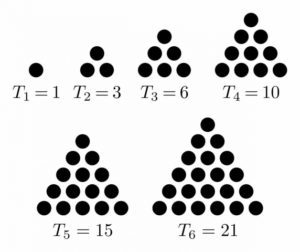Gnomonic growth is also seen with square root rectangles.  Starting with a square side = 1, each successive rectangle is built on the diagonal of the previous one to create squares of area 2, 3, 4 and 5.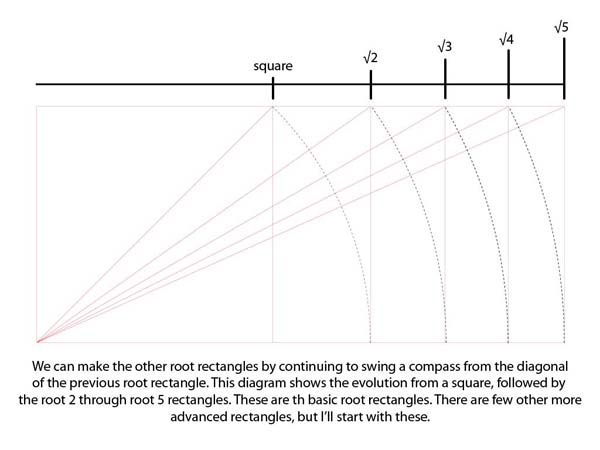And this is also found with the Fibonacci spiral.  Staring with a square we build new squares to create a spiral of squares which grows and grows by the Fibonacci sequence 1, 1, 2, 3, 5, 8, 13, 21, 34 and 55.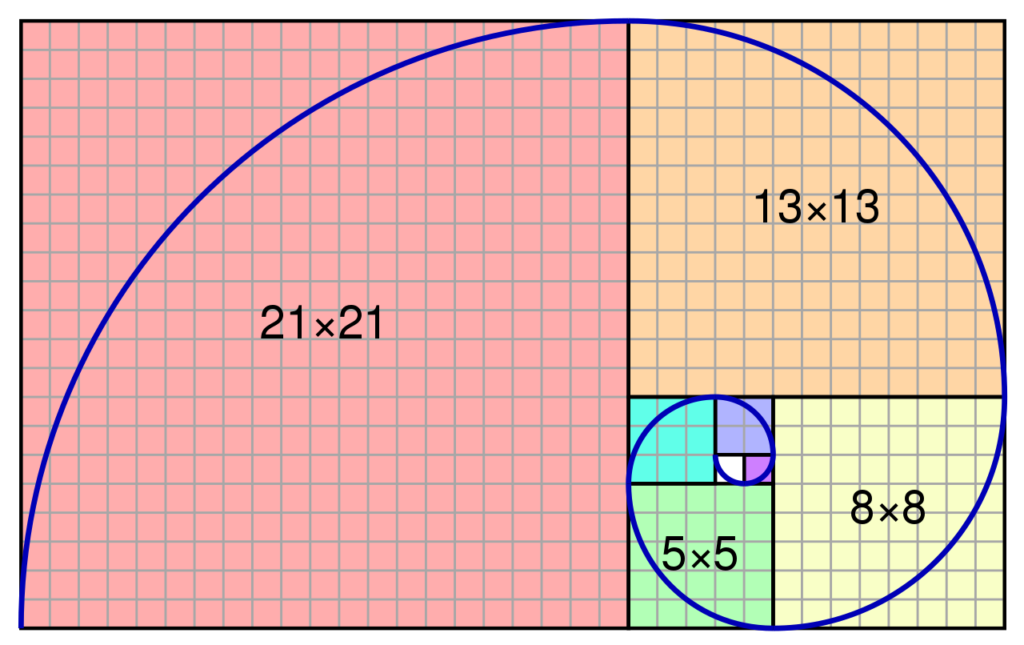“Contemplation of the gnomon leads to an understanding of one of nature’s most common principles, growth by accretion.”2

This is seen in the way bones, teeth, horns, and shells grow.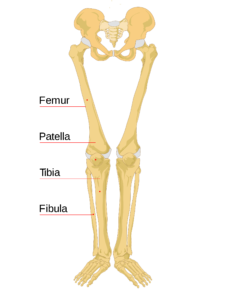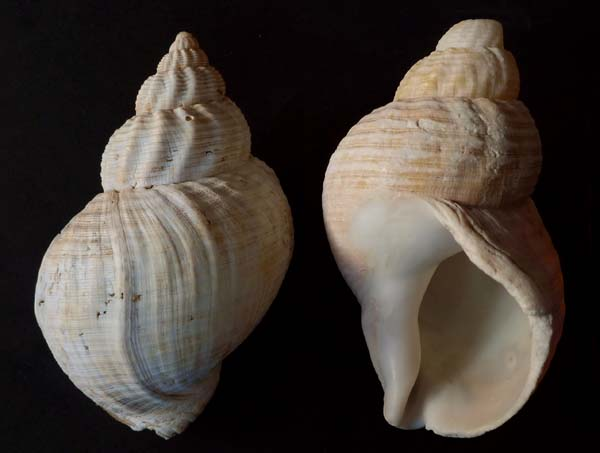They grow in size, increasing length and width, but maintain the same proportions.  This allows the center of gravity to remain the same throughout the course of its life.

Growth by accretion is also used by crystals, as it is the simplest law of growth.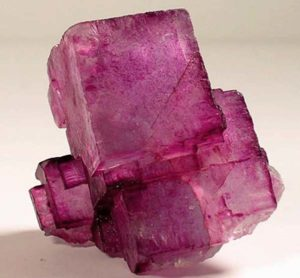# Fibonacci Circles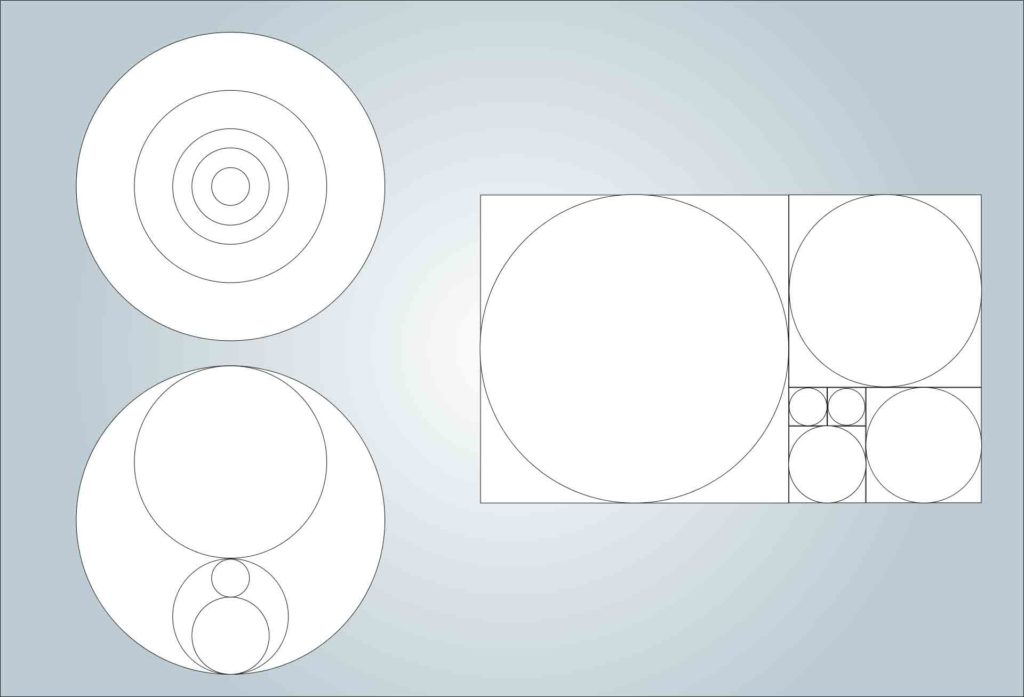The above circles are all in Fibonacci proportions.  These proportions of circles reveal interesting things.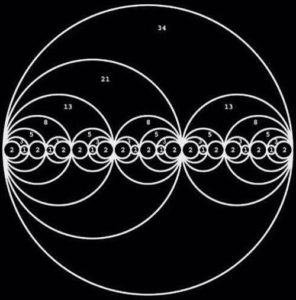Yin-Yang Fibonacci Circles.  Credit: Jain 108 Mathemagics: https://jain108.com/

See page 7 in Quantification by Scott Onstott and Construction Lesson #62.

Eight equally sized circles having diameters that are a term in the Fibonacci sequence will fit snugly around a central ninth circle if the ninth circle’s diameter is the next higher term in the sequence.

For example, eight circles with a diameter of 34 can fit around a central circle with diameter of 55, the next highest term in the Fibonacci sequence.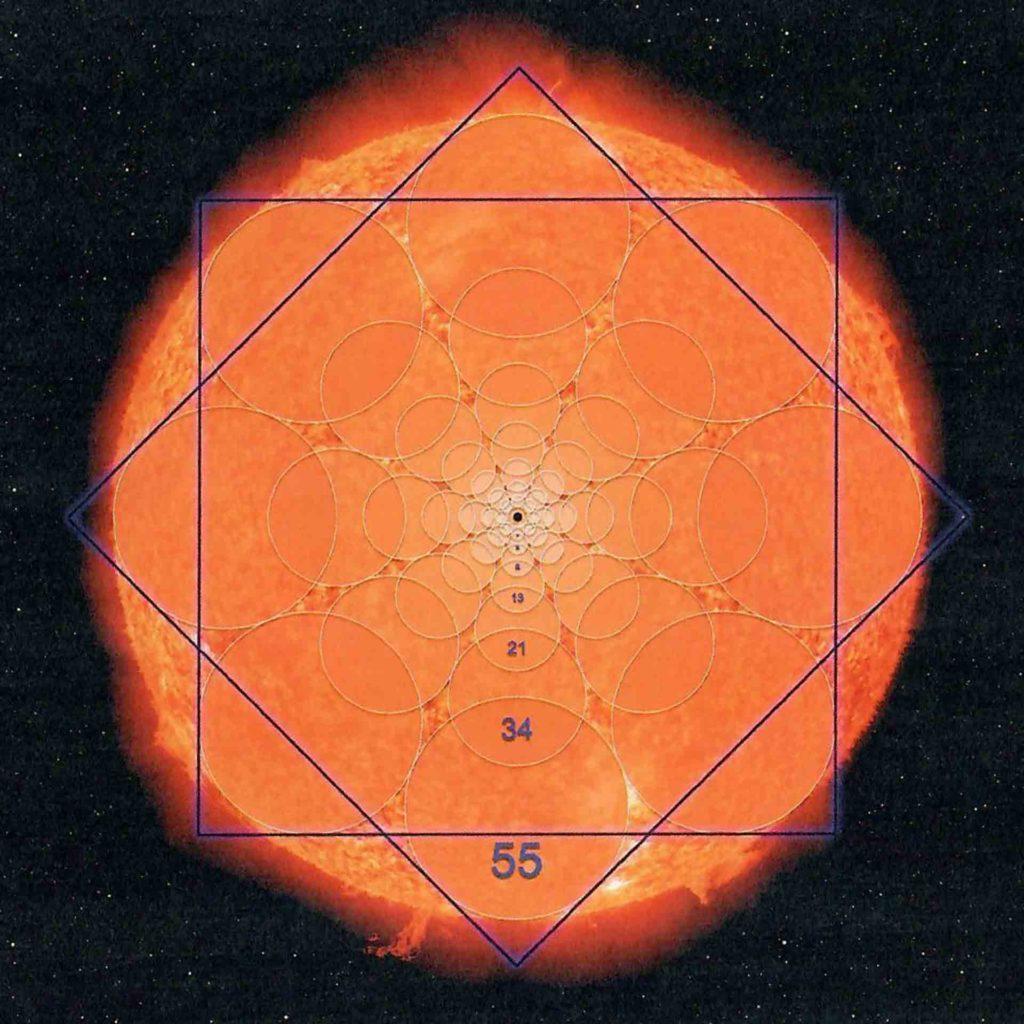Credit: Scott Onstott – Quantification

# Fibonacci Roots

Digital roots are a single-digit value obtained through an iterative process of summing digits.  We have seen this before in the Platonic solids, Sacred Number Canon and reducing to 9.

For example: 25920 = 2+5+9+2+0 = 18 = 1+8 = 9.

Thus, the digital root of 25920 is 9.

As we saw in the previous article, the digital roots in the Fibonacci sequence repeat infinitely with a period of 24.

“The Fibonacci sequence also has a pattern that repeats every 24 numbers.  This repetitive pattern involves a simple technique called numeric reduction in which all the digits of a number are added together until only 1 digit remains.  As an example, the numeric reduction of 256 is 4 because 2 + 5 + 6 = 13 and 1 + 3 = 4.  Applying numeric reduction to the Fibonacci series produces an infinite series of 24 repeating digits:

1, 1, 2, 3, 5, 8, 4, 3, 7, 1, 8, 9, 8, 8, 7, 6, 4, 1, 5, 6, 2, 8, 1, 9.”3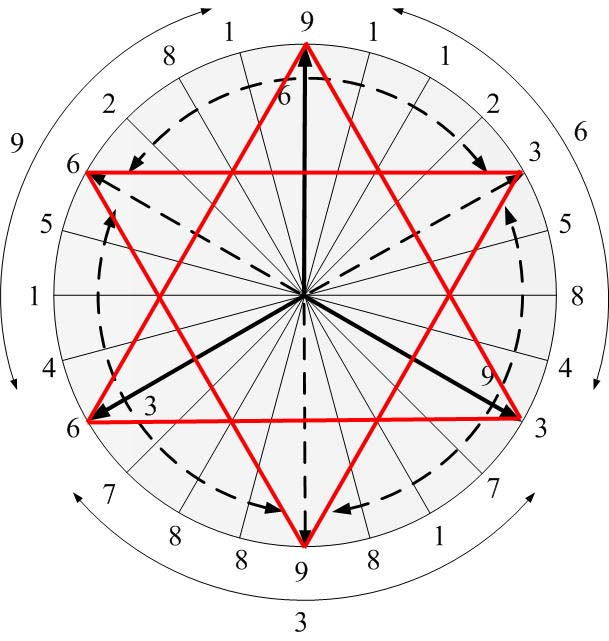When arranged in a circle, all 3, 6 and 9s are at the points of the two opposing equilateral triangles.

Every root arrayed around the circle added to its opposite root (180º away) = 9.

# Golden Mean Calipers

Golden Mean Calipers are a mechanical device that will locate the golden mean automatically.

It is constructed on the frame of a pentagram.  Phi is automatically built into it.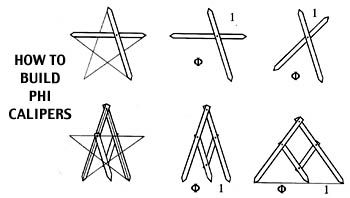“Mathematicians call this type of device a ‘linkage’ or a ‘pantograph’.  It is used for making enlargements or reductions of a drawing by phi proportions.”4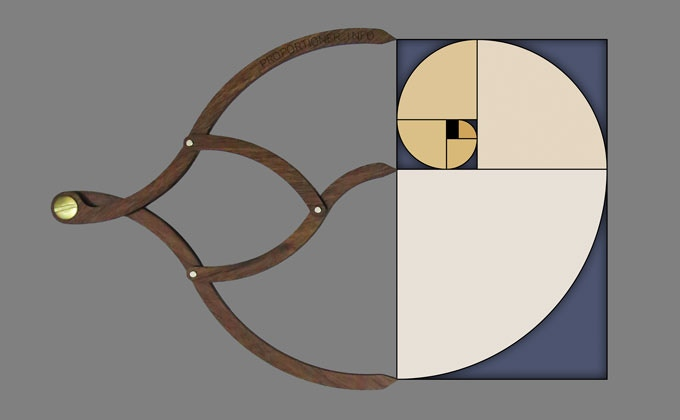It always shows a changing, relative phi relationship between pointers.

These calipers can be used to check the proportions on the diagrams creation in the Construction Lessons.

Reference Construction Lessons #63: The Golden Triangle & its Spiral.

# The Golden Triangle

We first saw the golden triangle in Article 31.

A golden triangle, or sublime triangle, is an isosceles triangle in which the duplicated side is in the golden ratio to the distinct side.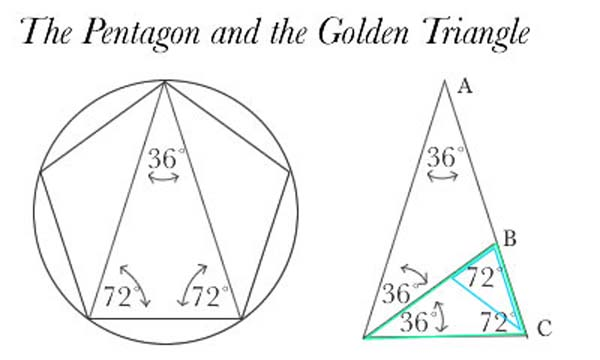In the case below, the ratio a:b is equivalent to the golden ratio φ.

This means if b = 1 then a = 1.618…

Also, b:a :: a: (a+b)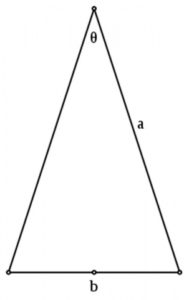Golden triangles are found in the nets of several stellations of dodecahedra and icosahedra.  They also show up in the 7-sided figure the Chestahedron, discussed in Article 67.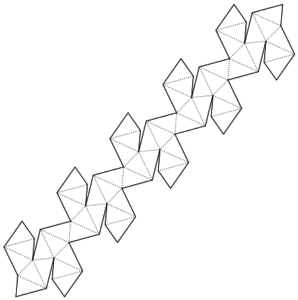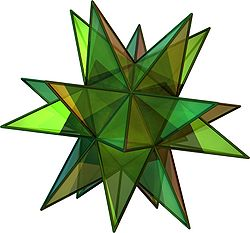The Great Stellated Dodecahedron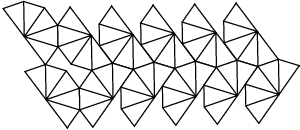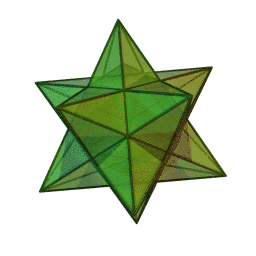The Small Stellated Dodecahedron

The golden triangle is also the shape of the triangles found in the points of pentagrams.  The vertex angle in this case is 36º and the base angles are 72º.

36 + 72 + 72 = 180.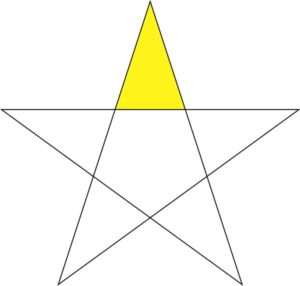The golden triangle can also be found in a decagon (10-sided polygon) by connecting any two adjacent vertices to the center.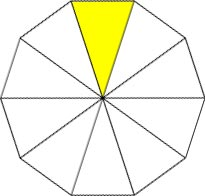The golden triangle is also the only triangle to have its three angles in a 2:2:1 proportion.

The golden triangle is also used to form a logarithmic spiral, as seen below.  “By bisecting the base angles, a new point is created that in turn, makes another golden triangle.  This bisection process can be continued infinitely, creating an infinite number of golden triangles.  A logarithmic spiral can be drawn through the vertices.”5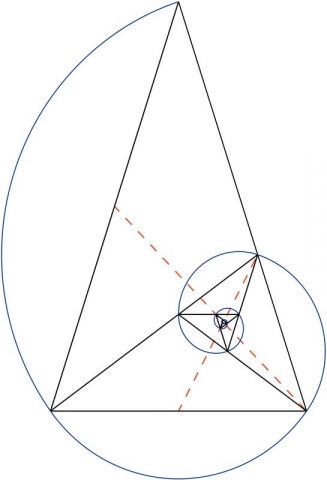# The Golden Gnomon

The Golden Gnomon is closely related to the golden triangle.  The golden gnomon is the obtuse triangle in which the ratio of the length of the equal (shorter) sides to the length of the third side is the reciprocal of the golden ratio.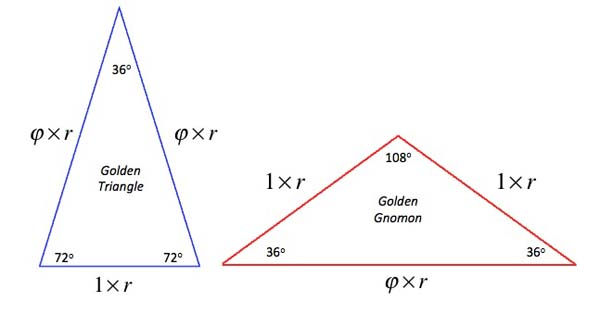The Golden Gnomon is also the only triangle to have its three angles in a 1:1:3 proportion.

The acute angles are 36°.  The apex angle is 108°.     (36 + 36 + 108 = 180)

The golden gnomon is the “roof” of a pentagon, as seen below.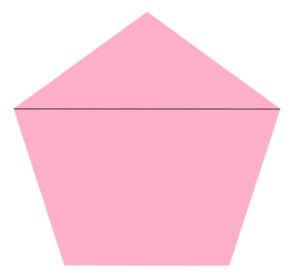“The golden triangle has a ratio of base length to side length equal to the golden section, whereas the golden gnomon has the ratio of side length to base length equal to the golden section.”6

A golden triangle can be bisected into a golden triangle and a golden gnomon.  The same is true for a golden gnomon.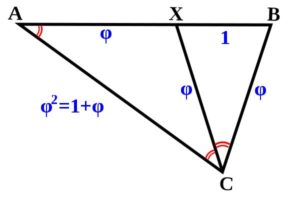Golden triangles self-replicate.

They whirl within and around one another, theoretically, to infinity.

Remember, every triangle in a pentagram is a golden triangle.

Scott Olsen tells us, “The arms of a pentagram contains the key to another golden section spiral as a continuous series of increasing or shrinking golden triangles.” 7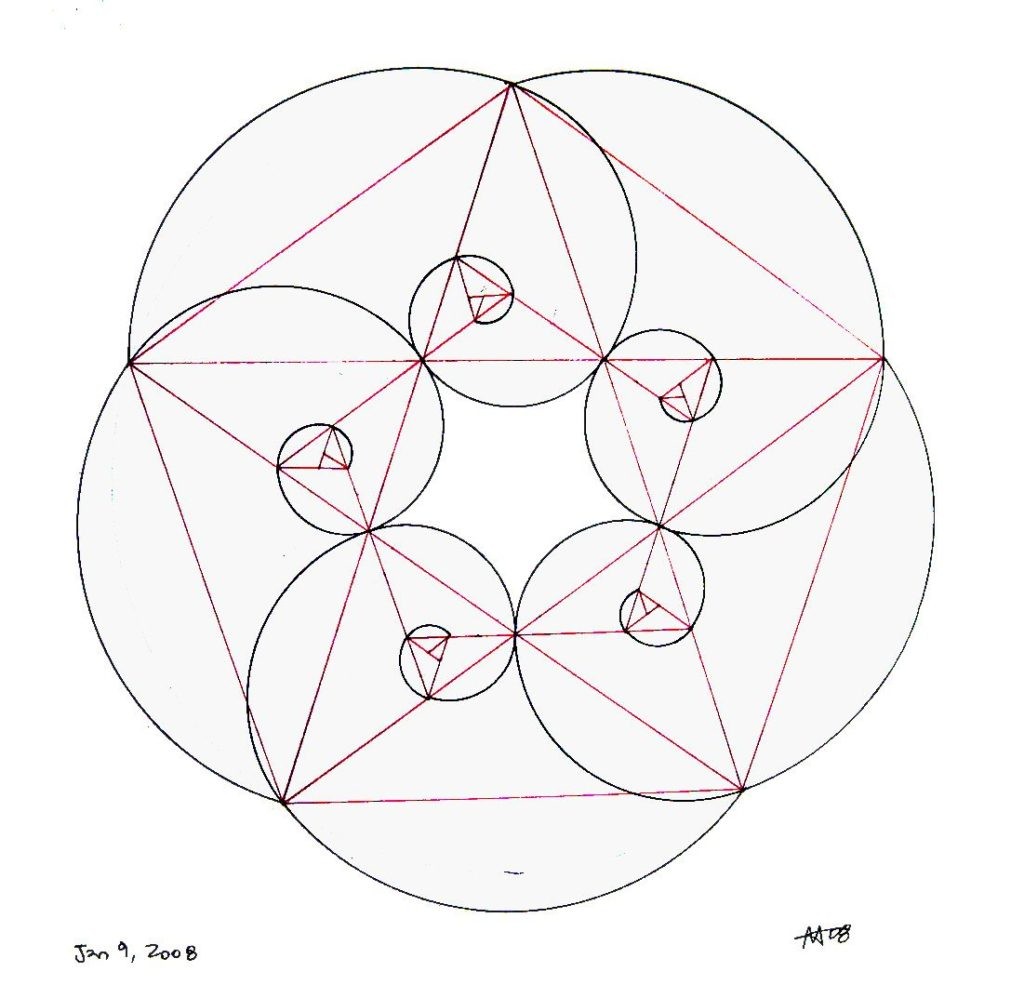# The Golden Rectangle

Freddy Silva writes in Secrets in the Fields, “Fundamental to the order of the Egyptian Freemasons were the Three Tables which represented the mathematical laws of the Universe:  The circle as the ethereal, the square as the transformation of the ethereal into matter, and the rectangle contained the proportions of the Golden Mean, and said to govern principles of sound and light.”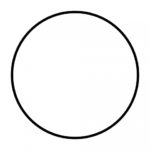A golden mean rectangle is a rectangle whose sides are in phi ratio.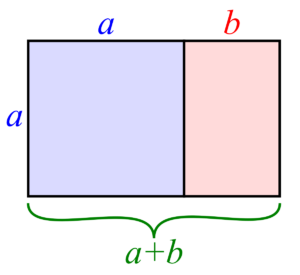If the short side = 1, the long side = Φ.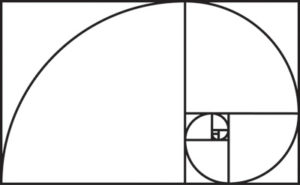Recall, an icosahedron’s 12 vertices are defined by 3 perpendicular golden section rectangles (pictured below).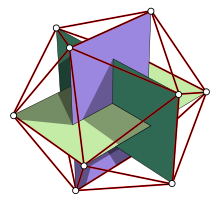12 of the dodecahedron’s 20 vertices are defined by 3 perpendicular Φ2 rectangles.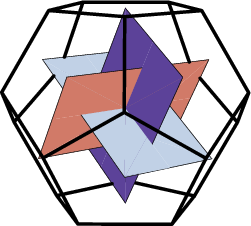The remaining 8 vertices are found by adding a cube of edge length Φ.

See page 153 in the Quadrivium.

Removing a square from a golden rectangle produces another smaller golden rectangle.

Adding a square to a golden rectangle produces another larger golden rectangle.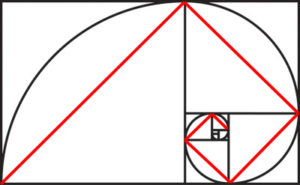In the golden rectangle, if the short side = 1 then the long side = Φ.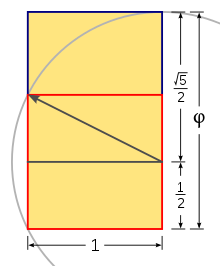Constructing a golden rectangle from a square

In other words, a circle drawn from the center of a square’s base touching the opposite corners of the square (radius r) will produce the golden section’s proportions along both sides of the extended baseline.  The total length of these reciprocal golden rectangles is 2.236 units, this number being identical with √5.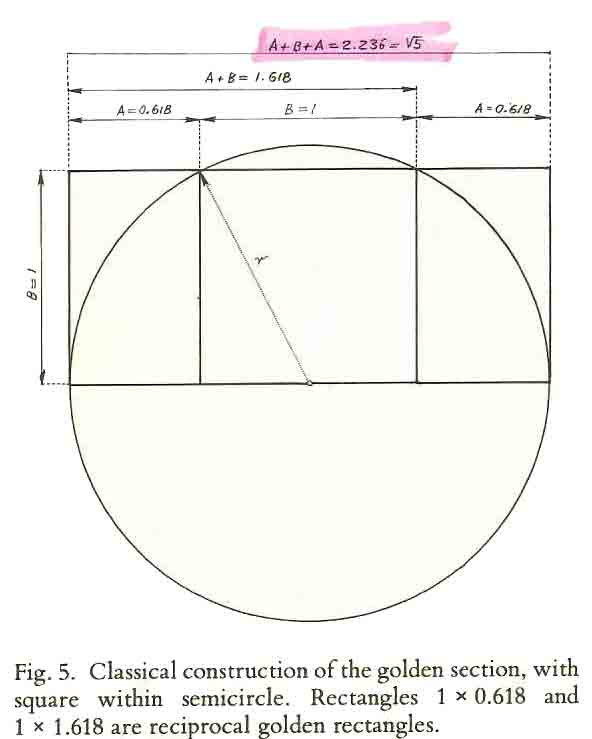Credit: Gyorgy Doczi – The Power of Limits, 1981

Golden rectangles are used widely in design:

furniture, clothing, pens, mirrors, windows, writing pads, pans with handles, logos, index cards, business cards, grocery bags, picture frames, TV sets, cassette tapes, stereo speakers, computer screens, smart phones, Georgian front doors…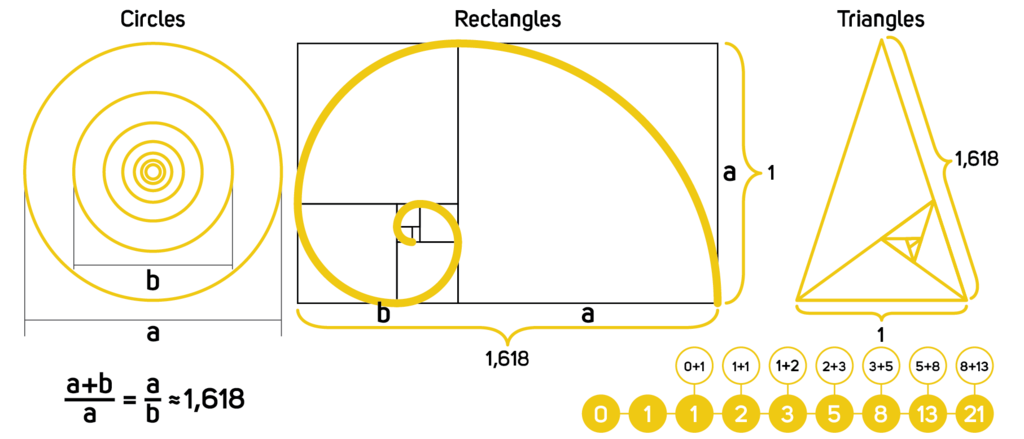Reference Construction Lesson #20: Root Rectangles & Phi to see how the golden ratio relates to the square root rectangles.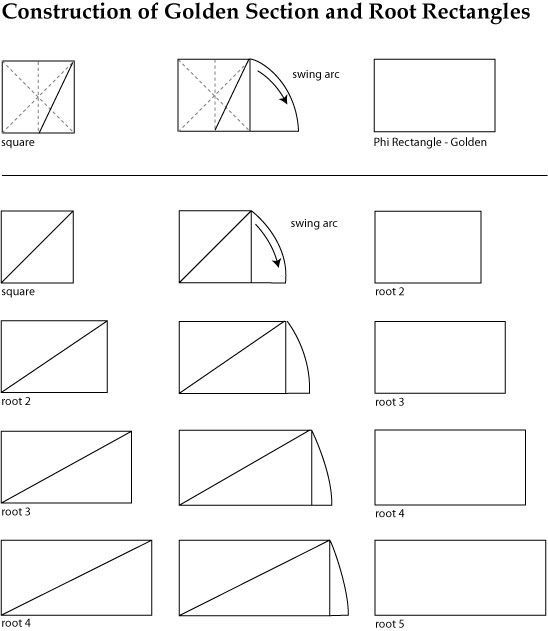Reference Construction Lesson #64: Constructing the Golden Rectangle and Pentagon Simultaneously

In this construction the basic figures of geometry expressing the number 5 are all displayed in one scheme.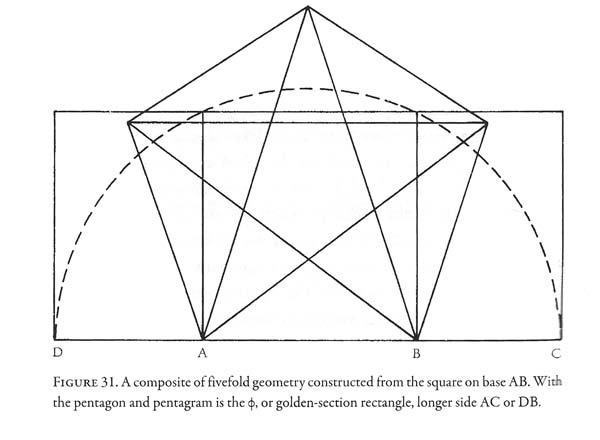Credit: John Michell

# Gustav T. Fechner (1801-1887) & Golden Rectangles

Gustav Fechner was a German physicist, psychologist, and philosopher.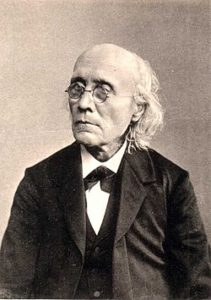He devised an experiment to give the ‘art’ of aesthetics a mathematical foundation.

He statistically measured aesthetic preferences using a series of 10 rectangles and asked which was the most pleasing.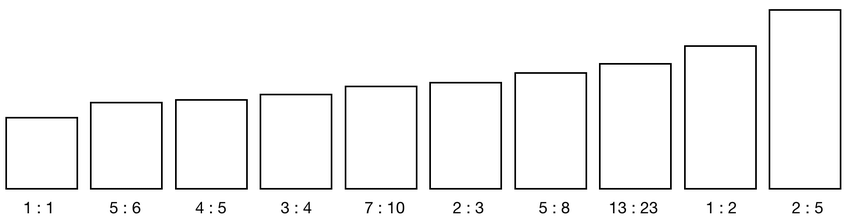He found people generally tend to prefer ratios and proportions closest to large consecutive Fibonacci numbers and the golden ratio.

His results have been verified by others since then.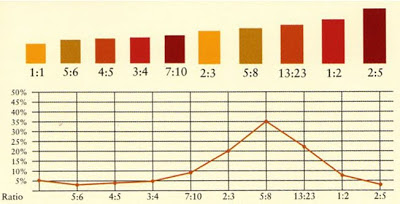Summary of Fechner’s results

“Phi’s self-replicating symmetry appeals to us because we unconsciously sense its internal balance, recognizing in the harmony of phi relationships the harmony within ourselves.  Phi resonates with the core of life, reminding us of our own infinite depth and beauty.”8

# The Golden Angle

The Golden Angle is the phi proportion of a full circle (360º).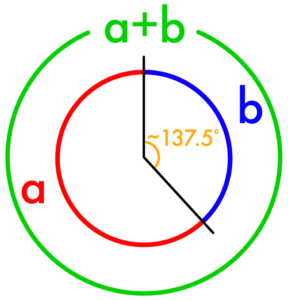The Golden Angle is thus:

360 degrees/Φ2

137.5 degrees = 1

225.5 degrees = Φ

This golden angle is seen in the phyllotaxis of plants.  This is covered extensively in Articles 178-187.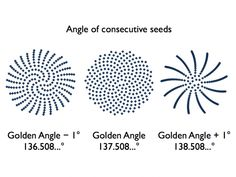In geometric analysis of plants, insects and animals another form of the ideal angle is often used.

In this case the supplementary angle of 137.5° is used.  This would be 180 – 137.5 = 42.5, which is often rounded off to 42°.

Hence both 42° and its complementary angle of 48° are used to analyze the geometry of life forms.  This means that the angles of 42°, 48°, 137.5° and 225.5° are all related to the golden ratio.

This is extensively illustrated in Samuel Colman’s Nature’s Harmonic Unity as is seen in subsequent articles.

1. http://www.cosmometry.net/phi-scaling-angle
2. Lundy, Miranda, Sacred Geometry
3. Meisner, Gary B., The Golden Ratio: The Divine Beauty of Mathematics, Race Point Publishing, 2018
4. Schneider, Michael, A Beginner’s Guide to Constructing the Universe, Harper Perennial, 1994
5. https://en.wikipedia.org/wiki/Golden_triangle_(mathematics)
6. ibid.
7. Olsen, Scott, The Golden Section: Nature’s Greatest Secret, Walker Books, 2006
8. Schneider, Michael, A Beginner’s Guide to Constructing the Universe, Harper Perennial, 1994